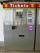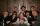Certificate

There is 31 students in a class. From mathematics was'nt worse mark than 2. Average mark in mathematics was 2.

How many students have mark 1 and how many mark 2?

Result

n(1) =  0
n(2) =  31

Solution:(1*a+2*b)/31 = 2
a+b=31

a+2b = 62
a+b = 31

a = 0
b = 31

Calculated by our linear equations calculator.

Leave us a comment of this math problem and its solution (i.e. if it is still somewhat unclear...):Be the first to comment!To solve this verbal math problem are needed these knowledge from mathematics:

Looking for help with calculating arithmetic mean? Looking for a statistical calculator? Do you have a system of equations and looking for calculator system of linear equations?

Next similar math problems:

1. Hotel roomsIn the 45 rooms, there were 169 guests, some rooms were three-bedrooms and some five-bedrooms. How many rooms were?
2. Classs meanClass size is 30 students, math was not worse than sign 2. Determine the number of pupils who were sign 1 if the class had a 1.4 sign average.
3. Three brothersThe three brothers have a total of 42 years. Jan is five years younger than Peter and Peter is 2 years younger than Michael. How many years has each of them?
4. TheatroTheatrical performance was attended by 480 spectators. Women were in the audience 40 more than men and children 60 less than half of adult spectators. How many men, women and children attended a theater performance?
5. Magic numberThe number 135 split to two addends so that one addend was 30 greater than 2/5 the addend.
6. Equations - simpleSolve system of linear equations: x-2y=6 3x+2y=4
7. Waiting roomIn the waiting room are people and flies. Together they have 15 heads and 50 legs (fly has 6 legs). How many people and flies are in the waiting room?
8. MushroomsEva and Jane collected 114 mushrooms together. Eve found twice as much as Jane. How many mushrooms found each of them?
9. AverageThe arithmetic mean of the two numbers is 71.7. One number is 5. Calculate the second number.
10. Linear systemSolve this linear system (two linear equations with two unknowns): x+y =36 19x+22y=720
11. The dormitoryThe dormitory accommodates 150 pupils in 42 rooms, some of which are triple and some are quadruple. Determine how many rooms are triple and how many quadruples.
12. Two numbersWe have two numbers. Their sum is 140. One-fifth of the first number is equal to half the second number. Determine those unknown numbers.
13. EquationsSolve following system of equations: 6(x+7)+4(y-5)=12 2(x+y)-3(-2x+4y)=-44
14. 5 people5 people have \$122000 and 1 person has \$539000 How much should each person (equally) pay?
15. TreesAlong the road were planted 250 trees of two types. Cherry for 60 CZK apiece and apple 50 CZK apiece. The entire plantation cost 12,800 CZK. How many was cherries and apples?
16. CoinsIn ticket maschine were together one hundred coins. They were only 20 and 50 cent coins. The sum total was 29 euros and 60 cents. How many were in ticket maschine coins and which type?
17. SistersSisters Janka and Danka are saved together 220 CZK. On a trip to Janka wants to take a fifth of her savings and Danka quarter. Then they will have 50 CZK on the trip. How much money saved Janka and Danka?